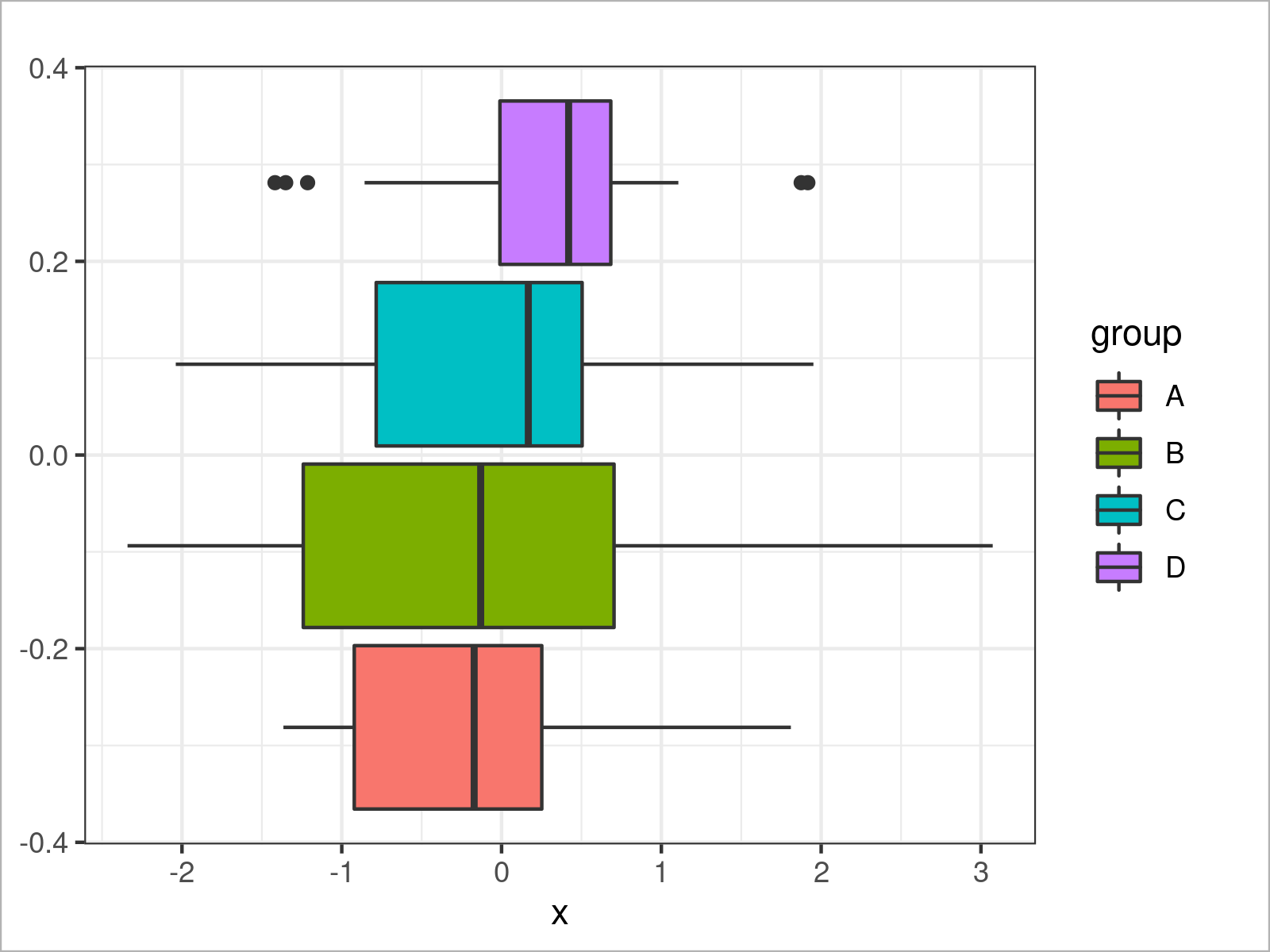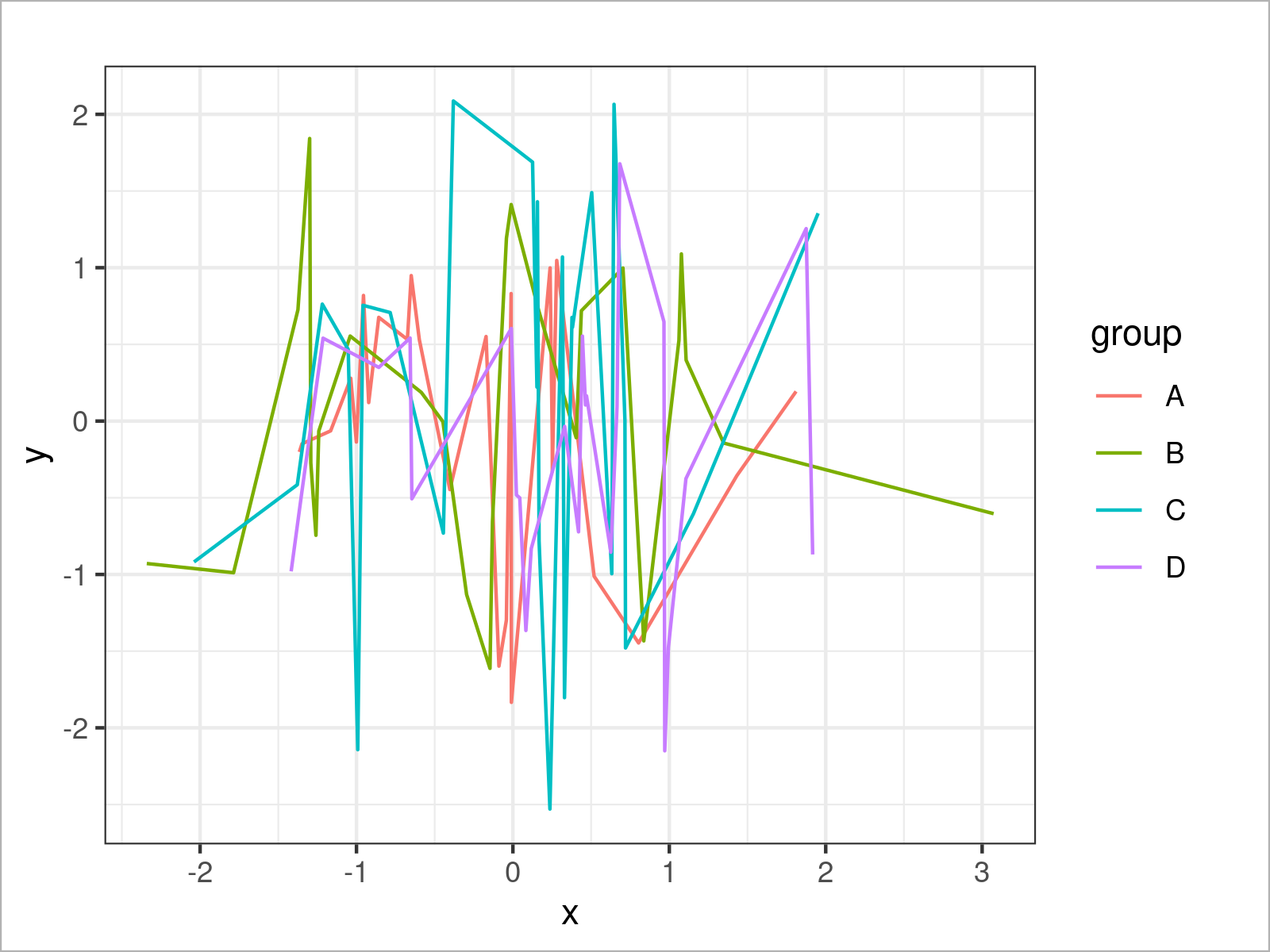# theme_bw ggplot2 Theme in R (6 Examples)

In this article you’ll learn how to change the ggplot2 theme to the theme_bw in the R programming language.

Here’s how to do it!

## Example Data & Add-On Packages

Have a look at the following example data.

```set.seed(926853)                           # Set random seed
data <- data.frame(x = rnorm(100),         # Create example data frame
y = rnorm(100),
group = LETTERS[1:4])Have a look at the previous table. It shows the first six rows of our exemplifying data, and that our data has three columns. The variables x and y have the numeric data type and the variable group is a character.

To be able to plot our data using the ggplot2 package, we also need to install and load ggplot2:

```install.packages("ggplot2")                # Install & load ggplot2
library("ggplot2")```

Now, we can draw different types of plots using the theme_bw theme style by applying the theme_bw() function as shown in the following examples.

## Example 1: Draw ggplot2 Scatterplot Using theme_bw()

```ggplot(data,                               # theme_bw scatterplot
aes(x = x,
y = y,
col = group)) +
geom_point() +
theme_bw()```## Example 2: Draw ggplot2 Density Plot Using theme_bw()

```ggplot(data,                               # theme_bw density plot
aes(x = x,
fill = group)) +
geom_density(alpha = 0.5) +
theme_bw()```## Example 3: Draw ggplot2 Histogram Using theme_bw()

```ggplot(data,                               # theme_bw histogram
aes(x = x,
fill = group)) +
geom_histogram(alpha = 0.5,
position = "identity",
bins = 50) +
theme_bw()```## Example 4: Draw ggplot2 Barchart Using theme_bw()

```ggplot(aggregate(y ~ group, data, sum),    # theme_bw barplot
aes(x = group,
y = y,
fill = group)) +
geom_bar(stat = "identity") +
theme_bw()```## Example 5: Draw ggplot2 Boxplot Using theme_bw()

```ggplot(data,                               # theme_bw boxplot
aes(x = x,
fill = group)) +
geom_boxplot() +
theme_bw()```## Example 6: Draw ggplot2 Line Plot Using theme_bw()

```ggplot(data,                               # theme_bw line plot
aes(x = x,
y = y,
col = group)) +
geom_line() +
theme_bw()```## Video & Further Resources

Would you like to know more about the ggplot2 package? Then you may have a look at the following video of my YouTube channel. I explain how to use the ggplot2 package in much more detail:

Please accept YouTube cookies to play this video. By accepting you will be accessing content from YouTube, a service provided by an external third party.If you accept this notice, your choice will be saved and the page will refresh.

On Statistics Globe, you can also find tutorials on how to plot different kinds of graphics such as xy-plots, density plots, barcharts, boxplots, histograms, and line plots using other ggplot2 themes:

Also, you can have a look at the introductions to other add-on packages that provide extra themes for ggplot2 plots:

Furthermore, you might have a look at the related tutorials on my homepage. A selection of tutorials is listed below:

To summarize: At this point you should have learned how to transform the ggplot2 theme to the theme_bw in R programming. If you have further questions, let me know in the comments.

Subscribe to the Statistics Globe Newsletter